• ## 带你深入理解矩阵乘法

万次阅读 多人点赞 2018-08-11 19:46:34
2、你已经学会了矩阵乘法，但如果你在计算矩阵乘法时还在使用“一行一列得一数”的方法，那我强烈建议你看看后面的内容。 因为，我将带你更加深刻地理解矩阵，与之而来是对矩阵乘法的全新计算方式。这不仅让你在...
为了不浪费大家宝贵的时间，开头我先简要说明一下这篇博文对哪些读者可能会有帮助
1、你是正在学习矩阵的乘法运算，觉得矩阵的乘法掌握起来很困难
2、你已经学会了矩阵乘法，但如果你在计算矩阵乘法时还在使用“一行乘一列得一数”的方法，那我强烈建议你看看后面的内容。
因为，我将带你更加深刻地理解矩阵，与之而来是对矩阵乘法的全新计算方式。这不仅让你在计算矩阵乘法时更快，而且更省心。

“矩阵就是数表”这可能是很多人第一次在线性代数课堂上听到的概念。我不能指责老师这样教有错，但这种肤浅的理解会给以后的学习带来越来越多的困难，无形之中让线性代数变得越来越“玄学”。
我认为好的学问都应该是通俗易懂的，因此让我们从一个最简单的概念开始——数数，或者说计量。
人类利用数字来表示事物的多少，但单有数字还是不够的，配合数字一起使用的还有一个概念——单位。例如，2L水，3kg钢铁等等。在更为抽象一点的事物上，还有与“单位”对应的一个概念——权。例如十进制数字 34 中的 4 的权是 1，而 3 的权是进制 10。简单来说，衡量任何实物，我们总是要现在此类事物中找一个看得顺眼的 “样品” 来作为衡量该类型其他事物的一个标准，看看需要衡量的事物是这个标准量的“几倍”，这样综合起来，我们心里就能有一个感性的认识。
认识一：其实没有标准，所有的标准都是由一个具体的事物（看得最顺眼的那个）来充当的。
上面的数数的例子都是单维度的，然而有很多量无法单单使用一对“数字+单位”这样的组合来描述清楚，而是需要多组。比如，平面上的点的位置需要两组，三维空间中的点的位置需要三组才能描述清楚，等等。而线性代数研究的就是空间。让我们还是再从最简单的概念开始——平面直角坐标系。
也许你会说，平面直角坐标系还不简单么，不就是这个么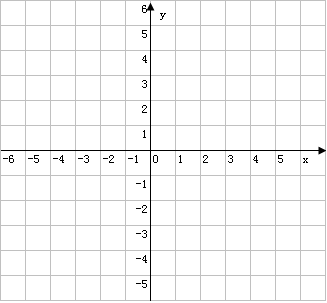但是，我更希望它在你心中样子长成这样：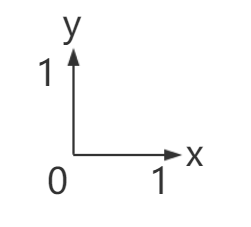官方的说法把它叫做“平面直角坐标系的基”，你可以把它理解为在平面中数点的位置时用到的“单位”（就叫它“标准1”吧）。点（2,3）实际上是：

很多时候我们看的顺眼事物往往就那一个，因此标准也是唯一的了。比如二维平面的标准：

或许有人会说，x方向的基并不一定需要和y方向上的基保持长度相同，夹角也不需要是直角（只要是两个方向就行了）。随便整一个：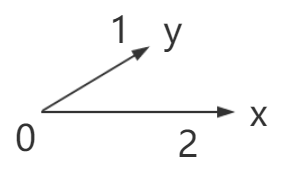这也能作为衡量平面中点的坐标的“单位”（暂且叫它“标准2”好了）。但让我们来看一个具体的例子：
如果有一个点在上面这个坐标系中的坐标是：x=3, y=4。我们是不是通常会“忍不住“计算一下：

这个算式很简单，但不知你想过没有，为什么我们要去算呢？为什么不就用

来表示这个点的坐标就好了呢，实际上，当我们写下第一个等号，在进行

这样的换算时，我们就已经放弃上面的“标准2”，又使用起了平面直角坐标系的“标准1”。
认识二：标准往往是唯一的，使用其他的标准时，我们会情不自禁地回到这个唯一的标准。而这个唯一的标准都有一个特性，你不会去想把它转化成其他标准。
而这个的简单算式，实际上就是一次“矩阵运算”！不信？让我们再来看一下这个简单的式子：

这就是简单而又直接的算法，我可没有用什么“一横乘一竖”。不过你应该能发现两种算法的答案居然是一样的！等等，你也许会说，这根本就不是矩阵的乘法，就算你这样变形着写，着充其量也就是个向量对矩阵的乘法。那下面再让我们来思考一个更为深刻的问题——矩阵究竟是什么？是数表么，当然不是。其实，上文已经对这个问题给出了答案，再看看 认知一 吧，标准是由事物来充当的；二维空间中衡量点的位置的标准是由二维点来充当的，n 维空间中衡量点的位置的标准是由 n 维点来充当的。更进一步的，n 维空间中衡量一个点的坐标需要描述清楚 n 个维度，那描述清楚每一个维度又需要什么呢？回到开篇所说，需要一个数，一个“单位”。而在 n 维空间中，一个“单位”，一个“标准”，就是由一个点来充当的啊。即，一个维度需要一个标准，一个标准就是一个点，n 个维度自然需要 n 个点，而这每个点又都是 n 维空间中的点，自然每个都有 n 个维度。n 个 n 维点，可不就是一个  的矩阵嘛。为什么长宽相等的矩阵出现频率这么高，因为这样的矩阵本质上是一个坐标系！一个描述 n 维空间的坐标系。
当你用一个点（专业的说法叫向量）乘以矩阵时，实际上是放弃了以这个矩阵作为坐标系来衡量这个点的位置，重新使用了我们看得最顺眼的衡量标准——“单位阵”；
当你用矩阵乘以矩阵时，如

实际上是进行了 n 次点乘以矩阵。你放弃了用矩阵 B 来衡量前面矩阵 A 中的 n 个点的位置，重新使用了我们看得最顺眼的衡量标准——“单位阵”。这矩阵A中的 n 个点在矩阵 B 中的位置就是矩阵 A 本身所描述的，在你放弃标准 B（进行了这次矩阵运算后），矩阵 A 中的 n 个点的位置变成由矩阵 C 来描述了。
最后，有了上面的铺垫，我们来看一下，在这样的理解下，矩阵乘法到底应该怎么算。

这里只有“横”，没有什么“竖”的概念。不要一个数一个数地算，应该一个点一个点的算。公式太抽象？举个栗子,要计算：

我们一行一行的算，第一行：

相信剩下两行的计算就不用我啰嗦了，大家都会。
相信大家在学线性代数的时候，一会儿是列向量，一会儿是行向量，叫人头晕，老师解释这只是种写法，并没有什么讲究，但事实又好像不是如此，因为你要“一行乘一列”嘛，前面放“行”后面放“列”，这式子没法算呐。但我在这里要呐喊：
认识三：从来就没有什么列向量，所有的向量都是行向量。
其实，你要是认为“从来就没有什么行向量，所有的向量都是列向量”也可以，那样的算法是一次算一列。事情的真相其实是所有的向量都是“同方向”的，你喜欢行向量就都是行向量好了，你喜欢列向量就都是列向量好了，反正他们不会同时存在。相信只有一个方向的向量会让你神清气爽，不再晕头转向。不过你要是用列向量的话，记得要从后往前算，有兴趣的读者可以研究一下具体的算法，如有不明之处可以下面留言交流。
最后再皮一下：其实我是喜欢都看成行向量的，一来写起来省纸又顺手，二来从左往右算，符合习惯。我还暗自揣测过为什么行比列顺手，可能跟书写工具有关吧，矩阵记号是西方发明的，西方人用硬笔，书写时沉腕，旋转肘关节自然横向方便，如果矩阵记号是中国人发明的，我们用软笔，书写时悬腕，旋转肩关节自然竖向方便，那说不定矩阵运算就是列运算更加方便了。
如果您觉得本篇文章对您有所帮助，那就支持我一下吧。展开全文线性代数 向量空间
• 矩阵乘法是个很高端的东西。 矩阵是什么 矩阵加减法 矩阵乘法 矩阵乘法的运算定律 模板 矩阵是什么？ 就是矩阵 矩阵加减法？ 矩阵加矩阵，就将对应的加上。 矩阵加常数，就将每个元素都加上这个...
矩阵乘法是个很高端的东西。  注意事项： 以下的讲述下标都从0开始

矩阵是什么矩阵加减法矩阵乘法
矩阵乘法的运算定律模板矩阵乘法的应用 例题一 斐波那契数列
描述
题目背景题目描述输入格式输出格式输入样例1输出样例1输入样例2输出样例2说明 解法代码 例题二 NOIP2013模拟联考14图形变换

DescriptionInputOutputSample InputSample OutputData ConstraintHint 解法代码 例题三 SDOI2009HH去散步
描述
题目描述输入格式输出格式输入样例1输出样例1说明 解法代码 总结补充

矩阵是什么？
不解释
矩阵加减法？
矩阵加矩阵，就将对应的加上。  矩阵加常数，就将每一个元素都加上这个常数。  减法同理。
矩阵乘法
一张好图，在这里发现的，方便理解矩阵乘法。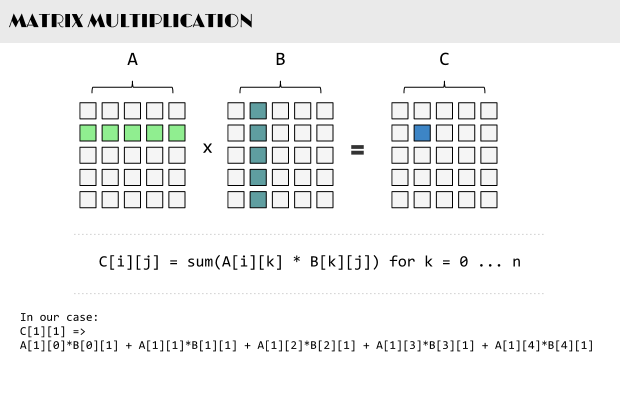一个

m∗n
$m*n$的的A矩阵，和一个

n∗p
$n*p$的B矩阵相乘，将得到一个

m∗p
$m*p$的矩阵C

C(i,j)=∑k=1nA(i,k)∗B(k,j)

C(i,j)=\sum_{k=1}^n A(i,k)*B(k,j)
可以简单地理解为，A中i行的元素，与B中j列的元素，对应相乘得到的和。

矩阵乘法的运算定律
（1） 不满足交换律  （2） 满足结合律：(AB)C=A(BC)  （3） 满足分配律：(A+B)C=AC+BC A(B+C)=AB+AC
模板
template <typename T,int M,int N>
struct Matrix
{
T mat[M][N];
inline Matrix(){memset(mat,0,sizeof mat);}
inline T* operator[](int i){return mat[i];}
};
template <typename T,int M,int N,int P>
inline Matrix<T,M,P> operator*(const Matrix<T,M,N>& x,const Matrix<T,N,P>& y)
{
Matrix<T,M,P> ret;
int i,j,k;
for (i=0;i<M;++i)
for (j=0;j<P;++j)
for (k=0;k<N;++k)
ret.mat[i][j]+=x.mat[i][k]*y.mat[k][j];
return ret;
}
template <typename T,typename T_>
inline T pow(T x,T_ y)
{
T ret=x;
--y;//这两句话可以忽略初值问题，但y<=0时就悲剧了
while (y)
{
if (y&1)
ret=ret*x;
y>>=1;
x=x*x;
}
return ret;
}
矩阵乘法的应用
矩阵乘法可以优化时间。  将某些操作转化为矩阵乘法的形式，就可以用快速幂减少时间。因为矩阵乘法满足结合律。

例题一 斐波那契数列
描述

题目背景
大家都知道，斐波那契数列是满足如下性质的一个数列：  • f(1) = 1  • f(2) = 1  • f(n) = f(n-1) + f(n-2) (n ≥ 2 且 n 为整数)
题目描述
请你求出 f(n) mod 1000000007 的值。
输入格式：
·第 1 行：一个整数 n
输出格式：
第 1 行： f(n) mod 1000000007 的值
输入样例#1：
5
输出样例#1：
5
输入样例#2：
10
输出样例#2：
55
说明
对于 60% 的数据： n ≤ 92  对于 100% 的数据： n在long long(INT64)范围内。

解法
这是一道裸斐波拉契数列。传统递推是O(N)的，显然会炸。  斐波拉契数列有个通项，但我们在这里用矩阵乘法解决。  我们知道f(n)是由f(n-1)和f(n-2)推过来的，不妨设一个1*2的矩阵

A=[f(n−2)f(n−1)]

A=\left[\begin{matrix}
f(n-2) & f(n-1)
\end{matrix}\right]
现在我们要通过A推出B

B=[f(n−1)f(n)]=[f(n−1)f(n−2)+f(n−1)]

B=\left[\begin{matrix}
f(n-1) & f(n)
\end{matrix}\right]=
\left[\begin{matrix}
f(n-1) & f(n-2)+f(n-1)
\end{matrix}\right]
我们要求出一个2*2的
转移矩阵 T，满足

A∗T=B

A*T=B
即

[f(n−2)f(n−1)]∗T=[f(n−1)f(n−2)+f(n−1)]

\left[\begin{matrix}
f(n-2) & f(n-1)
\end{matrix}\right]*T=\left[\begin{matrix}
f(n-1) & f(n-2)+f(n-1)
\end{matrix}\right]
我们知道，B=A B=A+A
对于T，A对B没有贡献，所以T=0
对于T，A对B有贡献，B=A*1，所以T=1
对于T，A对B有贡献，B=A*1+A*1，所以T=1
对于T，A对B有贡献，B=A*1+A*1，所以T=1
综上所述，

T=

T=\left[\begin{matrix}
0 & 1 \\
1 & 1
\end{matrix}\right]
显然这个式子是正确的。

[f(n−2)f(n−1)]∗=[f(n−1)f(n−2)+f(n−1)]

\left[\begin{matrix}
f(n-2) & f(n-1)
\end{matrix}\right]*\left[\begin{matrix}
0 & 1 \\
1 & 1
\end{matrix}\right]=\left[\begin{matrix}
f(n-1) & f(n-2)+f(n-1)
\end{matrix}\right]
求出这个矩阵后，就可以愉快地快速幂了。

时间复杂度O(lgN)

代码
#include <cstdio>
#include <cstring>
#include <algorithm>
using namespace std;
#define mod 1000000007
template <typename T,int M,int N>
struct Matrix
{
T mat[M][N];
inline Matrix(){memset(mat,0,sizeof mat);}
inline T* operator[](int i){return mat[i];}
};
template <typename T,int M,int N,int P>
inline Matrix<T,M,P> operator*(const Matrix<T,M,N>& x,const Matrix<T,N,P>& y)
{
Matrix<T,M,P> ret;
int i,j,k;
for (i=0;i<M;++i)
for (j=0;j<P;++j)
for (k=0;k<N;++k)
(ret.mat[i][j]+=x.mat[i][k]*y.mat[k][j])%=mod;
return ret;
}
template <typename T,typename T_>
inline T pow(T x,T_ y)
{
T ret=x;
--y;
while (y)
{
if (y&1)
ret=ret*x;
y>>=1;
x=x*x;
}
return ret;
}
int main()
{
long long n;
scanf("%lld",&n);
if (n==1)
putchar('1');
else
{
Matrix<long long,2,2> tmp;
tmp=tmp=tmp=1;
tmp=pow(tmp,n-1);
Matrix<long long,1,2> a;
a=1;//a=[f(0) f(1)]
a=a*tmp;//[f(0) f(1)]*(T^(n-1))=[f(n-1) f(n)]
printf("%lld\n",a);
}
}
例题二 【NOIP2013模拟联考14】图形变换

Description
翔翔最近接到一个任务，要把一个图形做大量的变换操作，翔翔实在是操作得手软，决定写个程序来执行变换操作。  翔翔目前接到的任务是，对一个由n个点组成的图形连续作平移、缩放、旋转变换。相关操作定义如下：  Trans(dx,dy) 表示平移图形，即把图形上所有的点的横纵坐标分别加上dx和dy；  Scale(sx,sy) 表示缩放图形，即把图形上所有点的横纵坐标分别乘以sx和sy；  Rotate(θ,x0,y0) 表示旋转图形，即把图形上所有点的坐标绕(x0,y0)顺时针旋转θ角度  由于某些操作会重复运行多次，翔翔还定义了循环指令：  Loop(m)  …  End  表示把Loop和对应End之间的操作循环执行m次，循环可以嵌套。
Input
第一行一个整数n(n<=100)表示图形由n个点组成；  接下来n行，每行空格隔开两个实数xi，yi表示点的坐标；  接下来一直到文件结束，每行一条操作指令。保证指令格式合法，无多余空格。
Output
输出有n行，每行两个空格隔开实数xi，yi表示对应输入的点变换后的坐标。  本题采用Special Judge判断，只要你输出的数值与标准答案误差不能超过1即可。
Sample Input
3  0.5 0  2.5 2  -4.5 1  Trans(1.5,-1)  Loop(2)  Trans(1,1)  Loop(2)  Rotate(90,0,0)  End  Scale(2,3)  End
Sample Output
10.0000 -3.0000  18.0000 15.0000  -10.0000 6.0000
Data Constraint
保证操作中坐标值不会超过double范围，输出不会超过int范围；  指令总共不超过1000行；  对于所有的数据,所有循环指令中m<=1000000；  对于60%的数据,所有循环指令中m<=1000；  对于30%的数据不含嵌套循环。
Hint
【友情提醒】  pi的值最好用系统的值。C++的定义为：#define Pi M_PI  Pascal就是直接为：pi  不要自己定义避免因为pi带来的误差。

解法
旋转公式：

绕(a,b)逆时针转θx′=(x−a)∗cosθ−(y−b)∗sinθ+ay′=(x−a)∗sinθ+(y−b)∗cosθ+b

绕(a,b)逆时针转\theta
\\
x'=(x-a)*cos\theta-(y-b)*sin\theta+a
\\
y'=(x-a)*sin\theta+(y-b)*cos\theta+b
暴力铁定超时。
先考虑使用2*2的转移矩阵T，但是只能对付乘法，加法和旋转就要用矩阵加法了。矩阵乘法和矩阵加法混在一起很麻烦（矩阵套矩阵？）。
我们可以新增一个常数项

A=[xy1]

A=\left[\begin{matrix}
x & y & 1
\end{matrix}\right]
现在要求出T1、T2、T3满足

[xy1]∗T1=[x+ay+b1][xy1]∗T2=[x∗ay∗b1][xy1]∗T3=[(x−a)∗cosθ−(y−b)∗sinθ+a(x−a)∗sinθ+(y−b)∗cosθ+b1]

\left[\begin{matrix}
x & y & 1
\end{matrix}\right]*T1=\left[\begin{matrix}
x+a & y+b & 1
\end{matrix}\right]
\\
\left[\begin{matrix}
x & y & 1
\end{matrix}\right]*T2=\left[\begin{matrix}
x*a & y*b & 1
\end{matrix}\right]
\\
\left[\begin{matrix}
x & y & 1
\end{matrix}\right]*T3=\left[\begin{matrix}
(x-a)*cos\theta-(y-b)*sin\theta+a
&
(x-a)*sin\theta+(y-b)*cos\theta+b
&
1
\end{matrix}\right]
这样加、乘就特别容易，将旋转公式用乘法分配律拆开，也可以得出转移矩阵

T1=⎡⎣⎢10a01b001⎤⎦⎥T2=⎡⎣⎢a000b0001⎤⎦⎥T3=⎡⎣⎢cosθ−sinθa−a∗cosθ+b∗sinθsinθcosθb−a∗sinθ−b∗cosθ001⎤⎦⎥

T1=\left[\begin{matrix}
1 & 0 & 0 \\
0 & 1 & 0 \\
a & b & 1
\end{matrix}\right]
\\
T2=\left[\begin{matrix}
a & 0 & 0 \\
0 & b & 0 \\
0 & 0 & 1
\end{matrix}\right]
\\
T3=\left[\begin{matrix}
\cos\theta & \sin\theta & 0 \\
-\sin\theta & \cos\theta & 0 \\
a-a*\cos\theta+b*\sin\theta & b-a*\sin\theta-b*\cos\theta & 1 \\
\end{matrix}\right]
可以代进去看看，是成立的。
于是我们可以将所有操作乘起来（有循环时递归，算出这个循环里一次的乘积后快速幂），最后在将每个坐标乘这个积，就是答案。

代码
英语不好，将theta打成xida，懒得改，注意一下就好了。
#include <cstdio>
#include <cstring>
#include <cmath>
#include <cassert>
#include <algorithm>
using namespace std;
#define I_O(x) freopen(""#x".in","r",stdin);freopen(""#x".out","w",stdout)
#define Pi 3.14159265358979323846
template <typename T,int M,int N>
struct Matrix
{
T mat[M][N];
inline Matrix(){memset(mat,0,sizeof mat);}
inline T* operator[](int i){return mat[i];}
};
template <typename T,int M,int N,int P>
inline Matrix<T,M,P> operator*(const Matrix<T,M,N>& x,const Matrix<T,N,P>& y)
{
Matrix<T,M,P> ret;
int i,j,k;
for (i=0;i<M;++i)
for (j=0;j<P;++j)
for (k=0;k<N;++k)
ret.mat[i][j]+=x.mat[i][k]*y.mat[k][j];
return ret;
}
template <typename T,typename T_>
inline T pow(T x,T_ y)
{
T ret=x;
--y;
while (y)
{
if (y&1)
ret=ret*x;
y>>=1;
x=x*x;
}
return ret;
}
int n;
Matrix<double,1,3> d;
char cz;
Matrix<double,3,3> dfs(int);
int main()
{
I_O(transform);
scanf("%d",&n);
int i;
double x,y;
for (i=0;i<n;++i)
{
scanf("%lf%lf",&d[i],&d[i]);
d[i]=1;
}
Matrix<double,3,3> a,tmp1,tmp2,tmp3;//分三个变量是为避免多次赋值
a=a=a=1;//a的初值
tmp1=tmp1=tmp1=1;
tmp2=1;
tmp3=1;
double xida,tmp_sin,tmp_cos;
int t;
while (scanf("%s",cz)==1)
{
switch (*cz)
{
case 'T':
//              1 0 0
//              0 1 0
//              a b 1
sscanf(cz,"Trans(%lf,%lf",&tmp1,&tmp1);
a=a*tmp1;
break;
case 'S':
//              a 0 0
//              0 b 0
//              0 0 1
sscanf(cz,"Scale(%lf,%lf",&tmp2,&tmp2);
a=a*tmp2;
break;
case 'R':
sscanf(cz,"Rotate(%lf,%lf,%lf",&xida,&x,&y);
xida=-xida/180*Pi;//顺时针角度转成逆时针弧度
//              cos           sin           0
//              -sin          cos           0
//              a-a*cos+b*sin b-a*sin-b*cos 1
tmp_sin=sin(xida);
tmp_cos=cos(xida);
tmp3=tmp_cos; tmp3=tmp_sin; tmp3=0;
tmp3=-tmp_sin; tmp3=tmp_cos; tmp3=0;
tmp3=x-x*tmp_cos+y*tmp_sin; tmp3=y-x*tmp_sin-y*tmp_cos;
a=a*tmp3;
break;
default:
sscanf(cz,"Loop(%d",&t);
a=a*dfs(t);
}
}
Matrix<double,1,3> tmp;
for (i=0;i<n;++i)
{
tmp=d[i]*a;
printf("%.4lf %.4lf\n",tmp,tmp);
}
}
Matrix<double,3,3> dfs(int t)
{
double x,y;
Matrix<double,3,3> a,tmp1,tmp2,tmp3;
a=a=a=1;
tmp1=tmp1=tmp1=1;
tmp2=1;
tmp3=1;
int times;
double xida,tmp_sin,tmp_cos;
for (scanf("%s",cz);*cz!='E';scanf("%s",cz))
{
switch (*cz)
{
case 'T':
sscanf(cz,"Trans(%lf,%lf",&tmp1,&tmp1);
//              1 0 0
//              0 1 0
//              a b 1
a=a*tmp1;
break;
case 'S':
sscanf(cz,"Scale(%lf,%lf",&tmp2,&tmp2);
//              a 0 0
//              0 b 0
//              0 0 1
a=a*tmp2;
break;
case 'R':
sscanf(cz,"Rotate(%lf,%lf,%lf",&xida,&x,&y);
xida=-xida/180*Pi;
//              cos           sin           0
//              -sin          cos           0
//              a-a*cos+b*sin b-a*sin-b*cos 1
tmp_sin=sin(xida);
tmp_cos=cos(xida);
tmp3=tmp_cos; tmp3=tmp_sin; tmp3=0;
tmp3=-tmp_sin; tmp3=tmp_cos; tmp3=0;
tmp3=x-x*tmp_cos+y*tmp_sin; tmp3=y-x*tmp_sin-y*tmp_cos;
a=a*tmp3;
break;
default:
sscanf(cz,"Loop(%d",&times);
a=a*dfs(times);
}
}
return pow(a,t);
}
例题三 【SDOI2009】HH去散步
描述

题目描述
HH有个一成不变的习惯，喜欢饭后百步走。所谓百步走，就是散步，就是在一定的时间 内，走过一定的距离。 但是同时HH又是个喜欢变化的人，所以他不会立刻沿着刚刚走来的路走回。 又因为HH是个喜欢变化的人，所以他每天走过的路径都不完全一样，他想知道他究竟有多 少种散步的方法。  现在给你学校的地图（假设每条路的长度都是一样的都是1），问长度为t，从给定地 点A走到给定地点B共有多少条符合条件的路径
输入格式：
第一行：五个整数N，M，t，A，B。其中N表示学校里的路口的个数，M表示学校里的 路的条数，t表示HH想要散步的距离，A表示散步的出发点，而B则表示散步的终点。  接下来M行，每行一组Ai，Bi，表示从路口Ai到路口Bi有一条路。数据保证Ai != Bi，但 不保证任意两个路口之间至多只有一条路相连接。 路口编号从0到N − 1。 同一行内所有数据均由一个空格隔开，行首行尾没有多余空格。没有多余空行。 答案模45989。
输出格式：
一行，表示答案。
输入样例#1：
4 5 3 0 0  0 1  0 2  0 3  2 1  3 2
输出样例#1：
4
说明
对于30%的数据，N ≤ 4，M ≤ 10，t ≤ 10。  对于100%的数据，N ≤ 50，M ≤ 60，t ≤ 2^30，0 ≤ A,B

解法
先说一下大意（我等好久才弄懂）。  给你一张图，要从A走到B，不能走上一次走的路，要走t步，问方案数。  不能走上一次走的路，一次。  举个例子：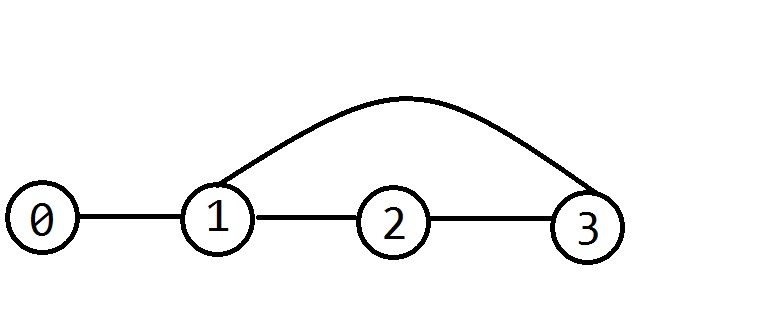路径可以这样：0->1->2->3->1->0  所以我们可以想到DP  设f[i][j]表示第i步走了j这条边的方案数，这样在转移时，就可以方便地判断，避免走上一次走的路。  转移：

f[i][bc]=∑ab≠bcf[i−1][ab]
$f[i][bc]=\sum_{ab\neq bc} f[i-1][ab]$  但是，我们发现，每次的决策点都是一样的，很明显能直接用矩阵乘法来转移，快速幂即可。
代码
#include <cstdio>
#include <cstring>
#include <cstdlib>
#include <algorithm>
using namespace std;
template <typename T,int M,int N>
struct Matrix
{
T mat[M][N];
inline Matrix(){memset(mat,0,sizeof mat);}
inline T* operator[](int i){return mat[i];}
};
template <typename T,int M,int N,int P>
inline Matrix<T,M,P> operator*(const Matrix<T,M,N>& x,const Matrix<T,N,P>& y)
{
Matrix<T,M,P> ret;
int i,j,k;
for (i=0;i<M;++i)
for (j=0;j<P;++j)
for (k=0;k<N;++k)
ret.mat[i][j]+=x.mat[i][k]*y.mat[k][j];
for (i=0;i<M;++i)
for (j=0;j<P;++j)
ret.mat[i][j]%=45989;//减少mod运算（mod运算很慢的）
return ret;
}
template <typename T,typename T_>
inline T pow(T x,T_ y)
{
T ret=x;
--y;
while (y)
{
if (y&1)
ret=ret*x;
y>>=1;
x=x*x;
}
return ret;
}
int n,m,t,u,v;
struct EDGE
{
int from,to;
EDGE* las;
EDGE* rev;
} e;
EDGE* last;
Matrix<long long,1,120> f;
Matrix<long long,120,120> T;
int li;//用于记录连接终点的边
int nl;
int main()
{
scanf("%d%d%d%d%d",&n,&m,&t,&u,&v);
int i,j=-1,x,y;
for (i=1;i<=m;++i)
{
scanf("%d%d",&x,&y);
++j;
e[j]={x,y,last[x],e+j+1};
last[x]=e+j;
if (y==v)
li[nl++]=j;
++j;
e[j]={y,x,last[y],e+j-1};
last[y]=e+j;
if (x==v)
li[nl++]=j;
}
EDGE* ei;
for (ei=last[u];ei;ei=ei->las)
f[int(ei-e)]=1;
m<<=1;
EDGE *ej,*end=e+m;
for (ei=e;ei<end;++ei)
for (ej=last[ei->to];ej;ej=ej->las)
if (ei->rev!=ej)
T[int(ei-e)][int(ej-e)]=1;//生成转移矩阵
f=f*pow(T,t-1);
int ans=0;
for (i=0;i<nl;++i)
ans+=f[li[i]];
printf("%d\n",ans%45989);
}
总结
遇到矩阵乘法的题，先将转移后的式子拆开，再帮它配转移矩阵。如果用普通的方式配矩阵必须出现加法，那么可以尝试加常数项上去。若有某些DP（还是递推？）的题目的决策点固定，那么可以在程序中生成转移矩阵，直接快速幂。
补充
矩阵乘法模板2.0
template <typename T,int M,int N>
struct Matrix
{
int m,n;
T mat[M][N];
inline Matrix(){m=M;n=N;memset(mat,0,sizeof mat);}
inline void SetUpSize(int _m,int _n){m=_m;n=_n;}
inline T* operator[](int i){return mat[i];}
};
template <typename T,int M,int N,int P>
inline Matrix<T,M,P> operator*(const Matrix<T,M,N>& x,const Matrix<T,N,P>& y)
{
Matrix<T,M,P> ret;
int i,j,k;
for (i=0;i<x.m;++i)
for (j=0;j<y.n;++j)
for (k=0;k<x.n;++k)
ret.mat[i][j]+=x.mat[i][k]*y.mat[k][j];
return ret;
}
template <typename T,typename T_>
inline T pow(T x,T_ y)
{
T ret=x;
--y;//这两句话可以忽略初值问题，但y<=0时就悲剧了
while (y)
{
if (y&1)
ret=ret*x;
y>>=1;
x=x*x;
}
return ret;
}
展开全文• 只有当左边的矩阵的列... 左矩阵一行乘以右矩阵一列(分别相乘,第一个数乘第一个数),乘完之后相加,即为结果的第一行一列的数 举个例子 比如现在我们有两个如下的矩阵 结果就是这样，我们得到了n*m的矩阵...
只有当左边的矩阵的列数等于右边矩阵的行数时,两个矩阵才可以进行矩阵的乘法运算  设两矩阵大小分别为

n∗q

n

∗

q

$n*q$和

q∗m

q

∗

m

$q*m$  相乘得到的矩阵大小为

n∗m

n

∗

m

$n*m$

左矩阵第一行乘以右矩阵第一列(分别相乘,第一个数乘第一个数),乘完之后相加,即为结果的第一行第一列的数

举个例子  比如现在我们有两个如下的矩阵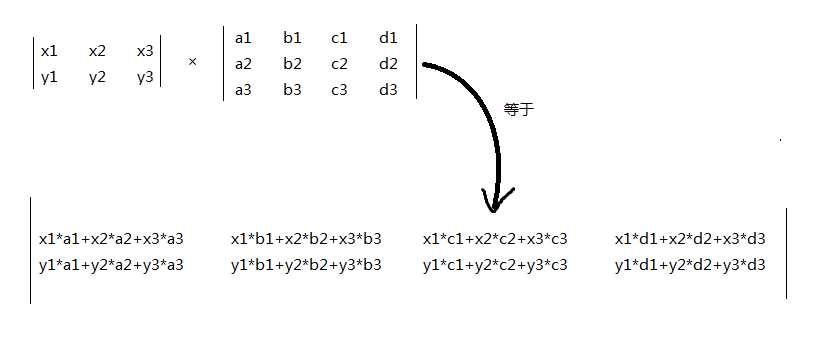结果就是这样，我们得到了n*m的矩阵
代码实现
写得丑
//by floatiy
//矩阵乘法模板

#include<iostream>
#include<cstdio>
using namespace std;
int n,m,p;//两矩阵分别为n*p,p*m
int a,b,c;
int main() {
cin>>n>>m>>p;
for(int i = 1; i <= n; i++) {
for(int j = 1; j <= p; j++) {
scanf("%d",&a[i][j]);
}
}
for(int i = 1; i <= p; i++) {
for(int j = 1; j <= m; j++) {
scanf("%d",&b[i][j]);
}
}
for(int i = 1; i <= n; i++) {
for(int j = 1; j <= m; j++) {
for(int k = 1;k <= p;k++){
c[i][j] += a[i][k] * b[k][j];
}
}
}
for(int i = 1;i <= n;i++){
for(int j = 1;j <= m;j++){
cout<<c[i][j]<<" ";
}
cout<<endl;
}
return 0;
}
展开全文• 问题描述 给定n个矩阵：A1,A2,...,An，其中Ai与Ai+1是可乘的，i=1，2...，n-1。确定计算矩阵连乘积的计算次序，使得依此次序计算矩阵连乘积需要的数乘次数最少。...在矩阵乘法中，第个矩阵的行数和第...
问题描述
给定n个矩阵：A1,A2,...,An，其中Ai与Ai+1是可乘的，i=1，2...，n-1。确定计算矩阵连乘积的计算次序，使得依此次序计算矩阵连乘积需要的数乘次数最少。

矩阵乘法的顺序安排
对于图像处理来说，矩阵运行是中必不可少的重要数学方法，另外在神经网络、模式识别等领域也有着广泛的用途。在这里就先来简单复习一下矩阵的相关知识：

矩阵乘法
在矩阵乘法中，第一个矩阵的行数和第二个矩阵的列数必须是相同的。先来看一个简单的例子：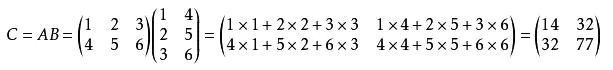之所以这样要求，是因为矩阵的乘法定义中，就要求了，第一个矩阵每一行和第二个矩阵每一列相对应位置的数字做乘的操作：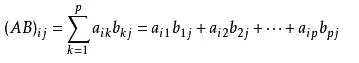如果A矩阵是p×q的矩阵，B是q×r的矩阵，那么乘积C是p×r的矩阵。它们一共计算了p×q×r次。
以下是一段计算两个矩阵乘积的标准算法：
void matrixMultiply(int[][] matrixA, int[][] matrixB,int[][] matrixC,int ra, int ca, int rb, int cb) {
if (ca != cb) {
System.err.println("矩阵不可乘！");
return;
}   // end if

for (int i = 0; i < ra; i++) {
for (int j = 0; j < cb; j++) {
int sum = matrixA[i] * matrixB[j];
for (int k = 0; k < ca; k++) {
sum += matrixA[i][k] * matrixB[k][j];
}   // end for
matrixC[i][j] = sum;
}
}
}


顺序安排
假设给定3个矩阵，A、B、C，它们的规模分别是10×100、100×5和5×50。
如果按照((AB)C)的顺序计算： 为计算AB（规模10×5），需要做10×100×5=5000次标量乘法，再与C相乘又需要做10×5×50=2500次标量乘法， 共需要7500次标量乘法。如果按照(A(BC))的顺序计算： 为计算BC（规模100×50），需要做100×5×50=25000次标量乘法，再与A相乘又需要做10×100×50=50000次标量乘法，共需要75000次标量乘法。
因此，按第一种顺序计算矩阵连乘要比第二种顺序快10倍。所以，进行一些计算来确定最有顺序还是值得的。

动态规划法
设mLeft,Right是进行矩阵乘法ALeftALeft+1···ARight-1ARight所需要的乘法次数。为一致起见，mLeft,Left=0。设最后的乘法是（ALeft···Ai）（Ai+1ARight），其中 Left ≤ i ≤ Right。
此时所用的乘法次数为：mLeft,i + mi+1,Right + cLeft-1cicRight。这三项分别代表计算（ALeft···Ai）、（Ai+1ARight）以及它们的乘积所需要的乘法。除了最后的答案，还要显示实际的乘法顺序，所以我们还要记录i的值，由此得到以下算法：
public static void optMatrix(int[] c, long[][] m, int[][] lastChange) {
int n = c.length - 1;

for (int left = 0; left < n; left++) {
m[left][left] = 0;
}
for (int k = 1; k <= n; k++) {
for (int left = 1; left <= n - k; left++) { // k is right - left，即子问题规模
// For each postion
int right = left + k;
m[left][right] = INFINITY;              // 置为无穷大
for (int i = left; i < right; i++) {    // i为断开位置
long thisCost = m[left][i] + m[i + 1][right] +
c[left - 1] * c[i] * c[right];

if (thisCost < m[left][right]) {    // Update min
m[left][right] = thisCost;
lastChange[left][right] = i;
}
}
}   // end inner for
}   // end outer for
}

这个程序包含三重嵌套循环，容易看出它以O(N3)时间运行。这里其实有更快地算法，但由于执行具体矩阵乘法的时间仍然很可能会比计算最有顺序的乘法的时间多得多，所以这个算法还是挺实用的。

欢迎转载，转载请注明出处！ 简书ID：@我没有三颗心脏

作者：我没有三颗心脏 链接：https://www.jianshu.com/p/1a5db71f1cbd 来源：简书 著作权归作者所有。商业转载请联系作者获得授权，非商业转载请注明出处。
展开全文算法
• 矩阵乘法：矩阵相乘最重要的方法是一般矩阵乘积。它只有在第个矩阵的数（column）和第二个矩阵的行数（row）相同时才有意义 。一般单指矩阵乘积时，指的便是  一般矩阵乘积。个m×n的矩阵就是m×n个数排成...矩阵 矩阵A+B
• ## MapReduce实现矩阵乘法

万次阅读 多人点赞 2014-10-10 11:05:22
在海量数据中淘金，已是各大互联网公司的既定目标，亚马逊是数据化运营的成功...所以将矩阵乘法移植到分布式系统中进行运算，可谓是基本需求，所以本文暂且从最基础开始，简单介绍使用MapReduce实现矩阵乘法的方式。mapreduce 分布式计算 矩阵 hadoop
• 一、矩阵乘法的五种方法 1.常规方法 如上图所示，所示的常规方法就是A矩阵的第行点乘B矩阵的第列，举个简单...其中矩阵A的第一行点乘矩阵第一列：、、、。 2.行方法（rows） 所谓的行方法就是我们对矩阵的乘...
• ## python 矩阵乘法

万次阅读 多人点赞 2019-02-09 13:55:54
python 矩阵乘法 python 矩阵有两种形式：array 和 matrix 对象（它们的区别在这里就不说了），下面介绍相关乘法 1. np.multiply 对 array 和 matrix 对象的操作相同 (1) a 和 b 维度相同 都是每对应元素相乘...
• ## 矩阵乘法的意义

万次阅读 2015-09-17 01:48:58
现举例子说明矩阵乘法的意义。如下图所示，个商店出售Beef pie，chicken pie，vegetable pie，其单价分别为3元，4元，2元。此外，还统计出了每天上述三种pie的售货量，求每天的总销售额。 我们可以创建...矩阵乘法 矩阵乘法实例 矩阵乘法意义 矩阵相乘
• 阅读后读者应能了解计算机算矩阵乘法与我们自己笔算有何不同，如何根据这些不同来设计最基本的矩阵乘法算法，并扩展成具有标准接口的函数，以及设计算法时值得注意之处。错漏之处欢迎指正。 1. 在计算机上arm
• 问题求解关于两个矩阵的乘积解题思路：参考线性代数里面的两个矩阵相乘的规则，我这里不再赘述，详情附上了个链接，我的编程也是用了里面的例子~~ [这里写链接内容]...编程
• ## 矩阵乘法总结

千次阅读 2019-05-09 19:52:55
矩阵是数的排列 矩阵 （这矩阵有2行和3列） 把矩阵与一个数相乘是容易的： 计算是这样的： ... 我们叫这个数 （"2"）为标量，所以这乘法被称为"标量乘法". ...求第一行和第一列的答案： "点积...
• 通过学习英伟达自带的例子matrixMul学CUDA库的使用。...这个例子是实现 C=A*B的矩阵相乘 // Use a larger block size for Fermi and above int block_size = 32; //original: dim3 dimsA(5*2*block_size, 5*2*bl
• 简介： 本文介绍cublasSgemm（）函数的使用。...本文，以个具体的矩阵乘法案例为例子，介绍cublasSgemm（）函数的使用。     正文： 我们以下图所示的矩阵运算为例进行讲解。     因为...c cuda
• ## 矩阵乘法复杂度分析

千次阅读 热门讨论 2020-12-27 17:38:12
背景 在很多机器学习或者数据挖掘论文中，里面或多或少的涉及到算法...算法复杂度在《数据结构》课程中也或多或少的涉猎，说完全不知道属于自己骗自己，简单的一些例子还是会分析的，但当涉及到复杂的目标方程矩阵复杂度
• 本文简单的描述了矩阵乘法的计算及矩阵运算(加法、数乘矩阵、矩阵乘法)在现实生活中对应的情况，说明数学是来源于生活，之所以与生活相差较大，只是因为在语言、符号演化过程中，数学进化的方向是趋向于抽象和一般。...矩阵 线性代数
• Python numpy 提取矩阵的某一行或某一列的实例如下所示：import numpy as npa=np.arange(9).reshape(3,3)aOut:array([[0, 1, 2],[3, 4, 5],[6, 7, 8]])矩阵的某一行aOut: array([3, 4, 5])矩阵的某一列a...
• ## 矩阵乘法优化DP

千次阅读 2016-06-04 17:03:35
矩阵乘法优化DP 在许多的DP题目中，转移方程本身不难推，但是需要循环的次数巨大。...这里只用十分简单的维DP做例子。如何乘在矩阵乘法中，两个矩阵A(a*b)和B(b*c)，相乘得到C(a*c)。 GDOI2015粗心的邮差
• 在我过往的认知中，矩阵乘法个函数，而且在这个函数中 向量每的值只与矩阵对应的有关 。也就是说b1只与a1j有关，bi只与aij有关。既然是函数，就存在 数据丢失 的可能，原本的向量b为k维，现在的向量是m维。m...
• ## MKL库矩阵乘法

千次阅读 2016-05-21 16:13:22
MKL库矩阵乘法 Posted on 2008年09月3日by cici1020 ...我再次来学术把。...我今天因为不想看3000多页的大文档，就在GOOGLE狂翻用Intel的Math Kernel ...关键词：Intel MKL MKL库 矩阵乘积 矩阵乘法 样例 例子
• 博主CUDA学习系列汇总传送门（持续更新）：编程...本质上看，矩阵陈发是向量内积的集合，CCC矩阵的每一个元素都是AAA矩阵一行和BBB矩阵一列的向量内积。 在C语言中，数据是按行存储的，所以BBB矩阵的访存不连续，导致.
• Toeplitz矩阵以及矩阵乘法FFT加速 1.Toeplitz矩阵 托普利兹矩阵，简称为T型矩阵，它是由Bryc、Dembo、Jiang于2006年提出的。托普利兹矩阵的主对角线上的元素相等，平行于主对角线的线上的元素也相等；矩阵中的各元素...算法
• 矩阵乘法怎么乘 设让矩阵a乘矩阵b得到矩阵c，那么c的第i第j个元素的值就等于a的第i与b的第j上对应元素相乘的和。 举个例子： (1234)∗(4321)=(abcd) \left( \begin{array}{} 1 &amp; 2 \\ 3 &...
• 按照鄙人的理解，矩阵乘法就是规定两个矩阵，取其中个矩阵的行数以及另个矩阵的数构成个新的矩阵，然后用被取的矩阵的对应去乘被取的矩阵的对应相乘然后再填到新矩阵的对应位置。其中涉及到的乘法...c语言
• ## tf矩阵乘法理解

千次阅读 2017-08-02 08:06:11
拿简单手写数字图像识别例子来说 个图像对应维数组[255,255,…]共784个元素 输出结果为0-9数字的十...w=nc b=cy=wx+bimport tensorflow as tf import numpy as np with tf.Session() as sess: #维向量tensorflow
• 对于3×33\times 3的例子，我们能够写出所有...我们现在引进矩阵符号来描述开始的系统，用矩阵乘法来描述计算步骤会更简单。注意三种不同类型的量都出现在例子中： Nine coefficientsThree unknownsThree right−h
• 背景 Python数据分析离不开矩阵的基础知识，周末看了章节的数学基础知识，重新学习了一下矩阵的乘法知识，线性代数的知识还是十年前上大学时学的，早就忘干净了，今日重新整理了一下，其实...Java实现矩阵乘法......